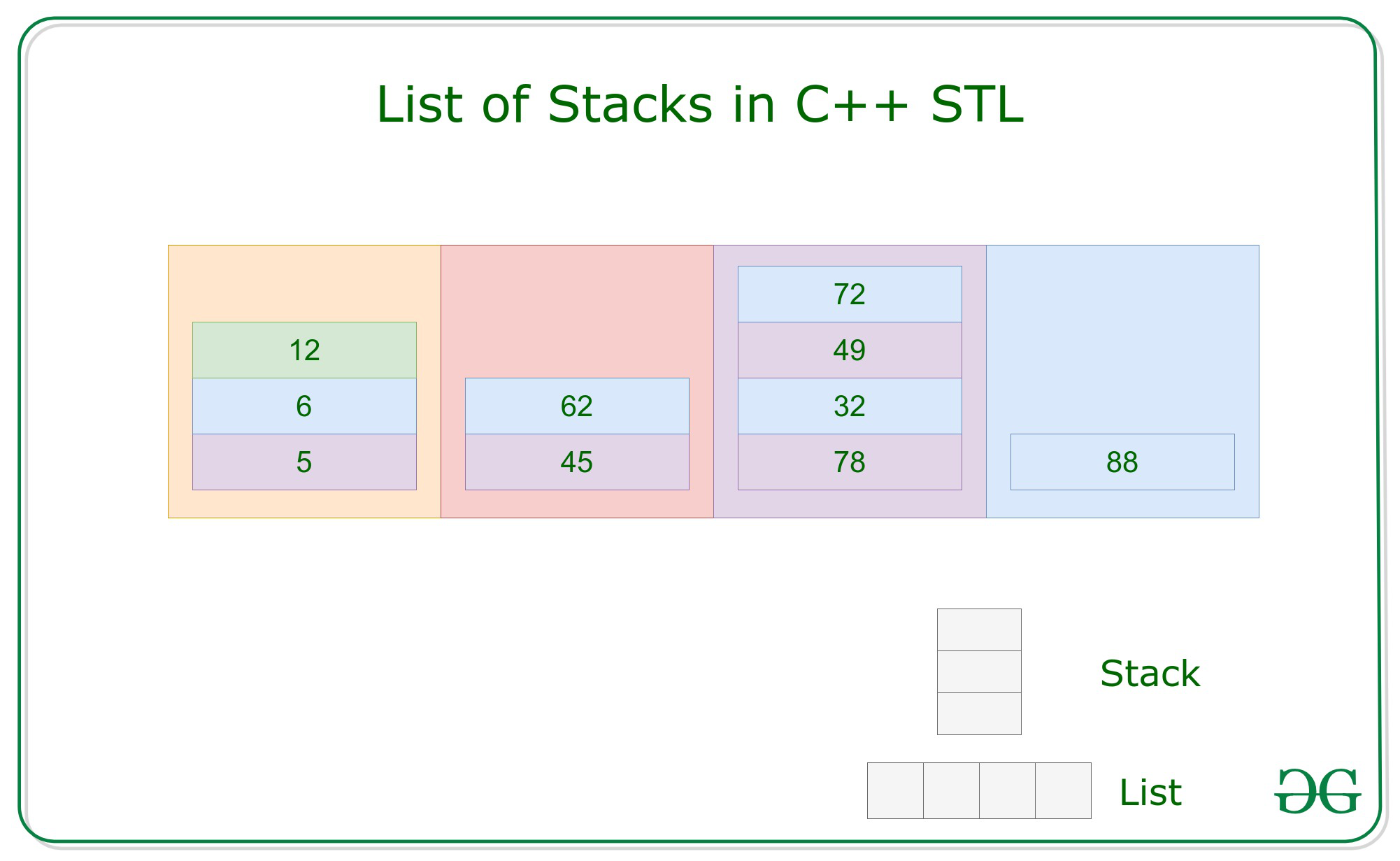# List of Stacks in C++ STL

Prerequisite: List, Stack

Lists are sequence containers that allow non-contiguous memory allocation. As compared to vector, list has slow traversal, but once a position has been found, insertion and deletion are quick.

Syntax:

list <Type> name_of_list;

Stack are a type of container adaptor with LIFO(Last In First Out) type of work, where a new element is added at one end and (top) an element is removed from that end only.

Syntax:

stack <Type> name_of_stack;

List of Stacks are a type of container which have a series of stacks, this is a two-dimensional container where N rows of list and M column of stacks, size of both dimension is non-fixed. which can be traversed and accessed using iterators.

Syntax:

list <stack <Type> > name_of_container(size);
where size is optionalExample:

list <stack <int> > ls(10);
size of list of stacks is 10

Insertion: Insertion in the list of the stack is done using the push() function.

Example:

## C++

 `// Loop to push element ` `// into a stack ` `for` `    ``i in[0, n] ` `    ``{ ` `        ``s.push(i) ` `    ``} ` ` `  `// Push Stack into the list ` `ls.push_back(s); `

Traversal: Traversal in a list of the stack is performed using iterators.

## C++

 `// Loop to iterate over list ` `for` `(iterator it = ls.begin(); ` `     ``it != ls.end(); it++) { ` ` `  `    ``// Stack of the List ` `    ``stack<``int``> ` `        ``st = *it; ` ` `  `    ``while` `(!st.empty()) { ` `        ``cout << st.top(); ` `        ``st.pop(); ` `    ``} ` `} `

Above code traverses list<int> ls at each index using starting iterators ls.begin() and ending iterator ls.end(). For accessing the element it uses (*it) as stack are pointers pointing to elements in list <stack <int> > ls.

Below is the program to illustrate the insertion and traversal in lists of stacks:

## C++

 `// C++ program for implementation ` `// of the lists of stack ` ` `  `#include ` `using` `namespace` `std; ` ` `  `// Function for printing the ` `// elements in a list ` `void` `showlist(list > ls) ` `{ ` ` `  `    ``// Traverse the list and ` `    ``// print row wise stack ` `    ``for` `(list >::iterator it1 ` `         ``= ls.begin(); ` `         ``it1 != ls.end(); ++it1) { ` ` `  `        ``// Copy rows in stack ` `        ``stack<``int``> it2 = *it1; ` ` `  `        ``// Print stack elements while ` `        ``// it is not empty ` `        ``while` `(!it2.empty()) { ` `            ``cout << it2.top() << ``" "``; ` `            ``it2.pop(); ` `        ``} ` `        ``cout << endl; ` `    ``} ` `} ` ` `  `// Driver Code ` `int` `main() ` `{ ` `    ``// List of stacks ` `    ``list > ls; ` ` `  `    ``// Insert rows in list ` `    ``for` `(``int` `i = 0; i < 10; ++i) { ` `        ``// Insert element in ` `        ``// stack as column ` `        ``stack<``int``> s; ` ` `  `        ``for` `(``int` `j = i; j < 10; j++) { ` `            ``s.push(j); ` `        ``} ` ` `  `        ``ls.push_back(s); ` `    ``} ` ` `  `    ``cout << ``"List of stack is : \n"``; ` `    ``showlist(ls); ` ` `  `    ``return` `0; ` `} `

Output:

```List of stack is :
9 8 7 6 5 4 3 2 1 0
9 8 7 6 5 4 3 2 1
9 8 7 6 5 4 3 2
9 8 7 6 5 4 3
9 8 7 6 5 4
9 8 7 6 5
9 8 7 6
9 8 7
9 8
9
```

Feeling lost in the world of random DSA topics, wasting time without progress? It's time for a change! Join our DSA course, where we'll guide you on an exciting journey to master DSA efficiently and on schedule.
Ready to dive in? Explore our Free Demo Content and join our DSA course, trusted by over 100,000 geeks!

Previous
Next